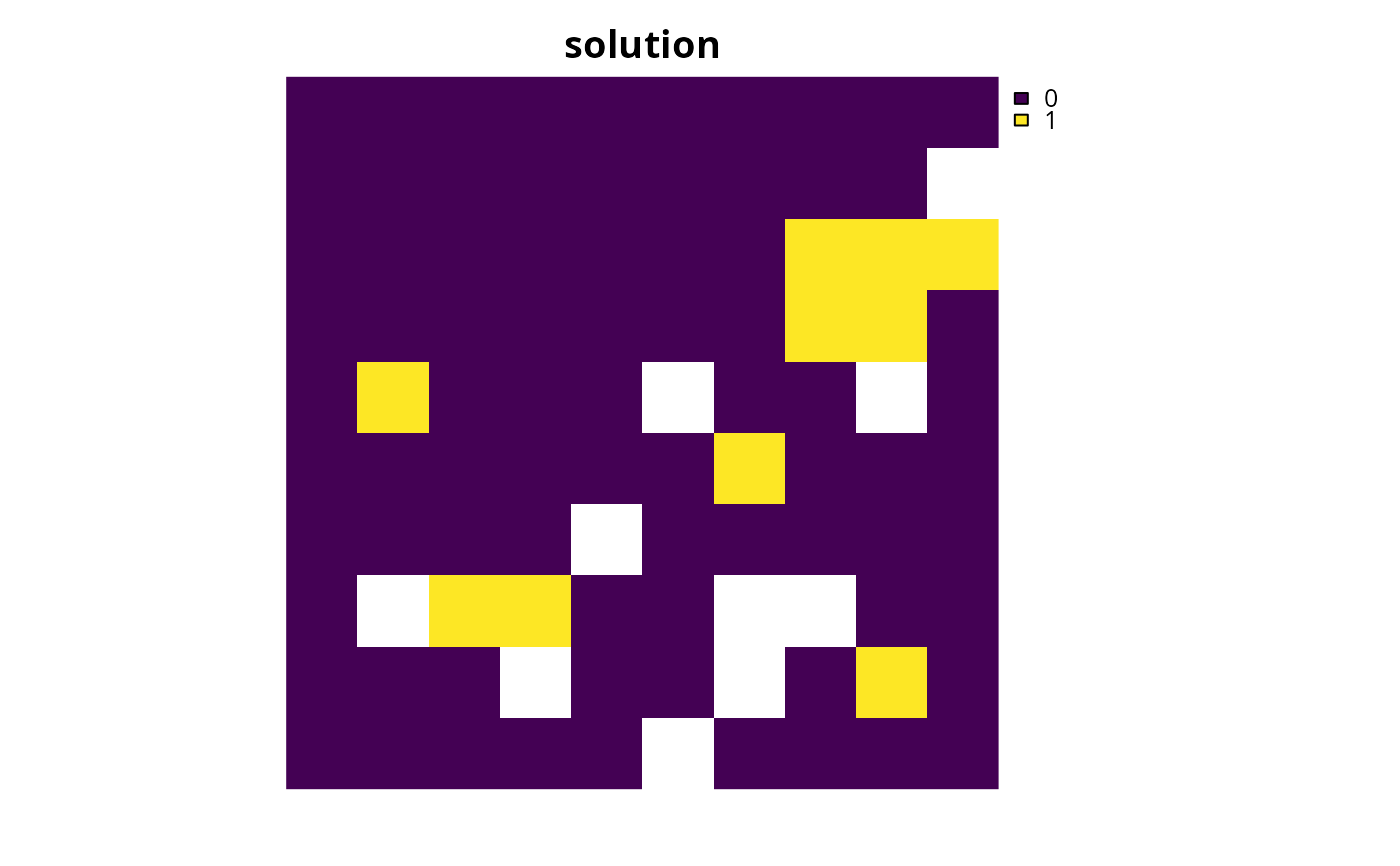Conservation planning problems that aim to maximize the representation of features given a budget often will not able to conserve all of the features unless the budget is very high. In such budget-limited problems, it may be desirable to prefer the representation of some features over other features. This information can be incorporated into the problem using weights. Weights can be applied to a problem to favor the representation of some features over other features when making decisions about how the budget should be allocated.

# S4 method for ConservationProblem,numeric

# S4 method for ConservationProblem,matrix
add_feature_weights(x, weights)

## Arguments

x

problem() (i.e., ConservationProblem) object.

weights

numeric or matrix of weights. See the Weights format section for more information.

## Value

Object (i.e., ConservationProblem) with the weights added to it.

## Details

Weights can only be applied to problems that have an objective that is budget limited (e.g., add_max_cover_objective()). They can be applied to problems that aim to maximize phylogenetic representation (add_max_phylo_div_objective()) to favor the representation of specific features over the representation of some phylogenetic branches. Weights cannot be negative values and must have values that are equal to or larger than zero. Note that planning unit costs are scaled to 0.01 to identify the cheapest solution among multiple optimal solutions. This means that the optimization process will favor cheaper solutions over solutions that meet feature targets (or occurrences) when feature weights are lower than 0.01.

## Weights format

The argument to weights can be specified using the following formats.

weights as a numeric vector

containing weights for each feature. Note that this format cannot be used to specify weights for problems with multiple zones.

weights as a matrix object

containing weights for each feature in each zone. Here, each row corresponds to a different feature in argument to x, each column corresponds to a different zone in argument to x, and each cell contains the weight value for a given feature that the solution can to secure in a given zone. Note that if the problem contains targets created using add_manual_targets() then a matrix should be supplied containing a single column that indicates that weight for fulfilling each target.

See penalties for an overview of all functions for adding penalties.

Other penalties: add_asym_connectivity_penalties(), add_boundary_penalties(), add_connectivity_penalties(), add_linear_penalties()

## Examples

# load ape package
require(ape)
#>
#> Attaching package: ‘ape’
#> The following objects are masked from ‘package:raster’:
#>
#>     rotate, zoom

data(sim_pu_raster, sim_features, sim_phylogeny, sim_pu_zones_stack,
sim_features_zones)

# create minimal problem that aims to maximize the number of features
# adequately conserved given a total budget of 3800. Here, each feature
# needs 20% of its habitat for it to be considered adequately conserved
p1 <- problem(sim_pu_raster, sim_features) %>%

# create weights that assign higher importance to features with less
# suitable habitat in the study area
(w2 <- exp((1 / cellStats(sim_features, "sum")) * 200))
#>   layer.1   layer.2   layer.3   layer.4   layer.5
#>  11.03585 606.63186  16.11015 108.65716  34.01731

# create problem using rarity weights

# create manually specified weights that assign higher importance to
# certain features. These weights could be based on a pre-calculated index
# (e.g., an index measuring extinction risk where higher values
# denote higher extinction risk)
w3 <- c(0, 0, 0, 100, 200)
# \dontrun{
# solve problems
s1 <- stack(solve(p1), solve(p2), solve(p3))

# plot solutions
plot(s1, main = c("equal weights", "rarity weights", "manual weights"),
axes = FALSE, box = FALSE)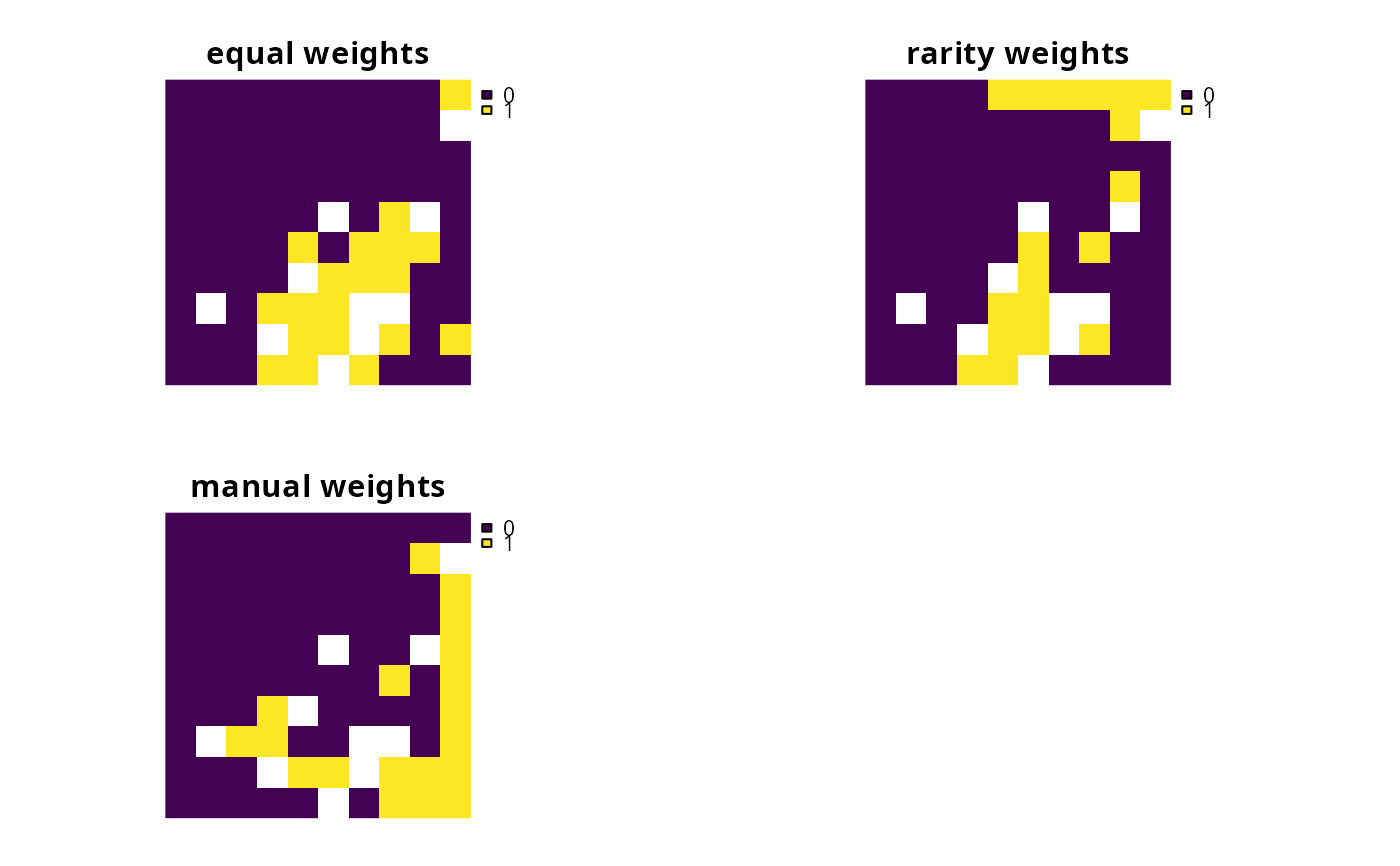# }

# plot the example phylogeny
# \dontrun{
par(mfrow = c(1, 1))
plot(sim_phylogeny, main = "simulated phylogeny")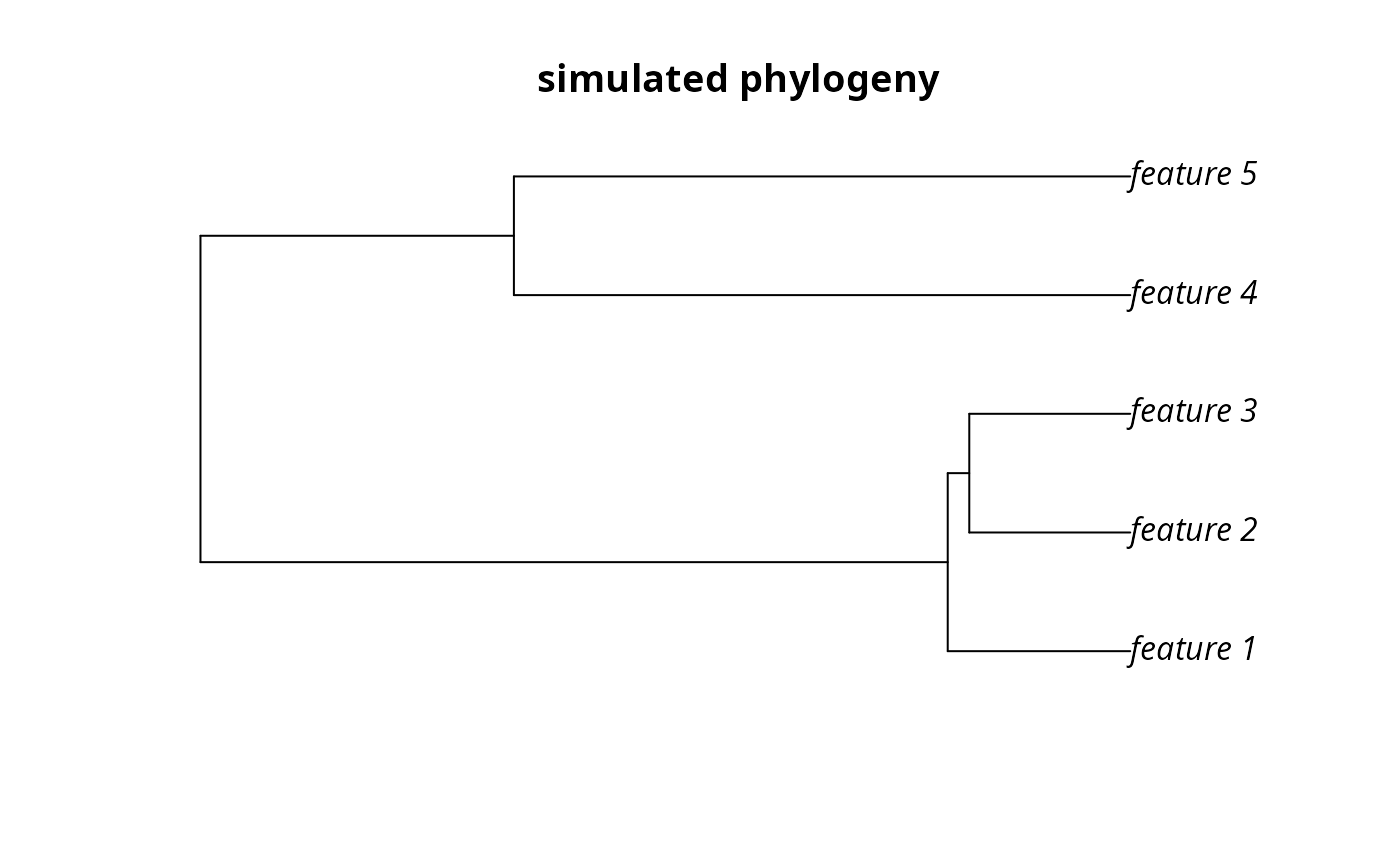# }
# create problem with a maximum phylogenetic diversity objective,
# where each feature needs 10% of its distribution to be secured for
# it to be adequately conserved and a total budget of 1900
p4 <- problem(sim_pu_raster, sim_features) %>%
# \dontrun{
# solve problem
s4 <- solve(p4)

# plot solution
plot(s4, main = "solution", axes = FALSE, box = FALSE)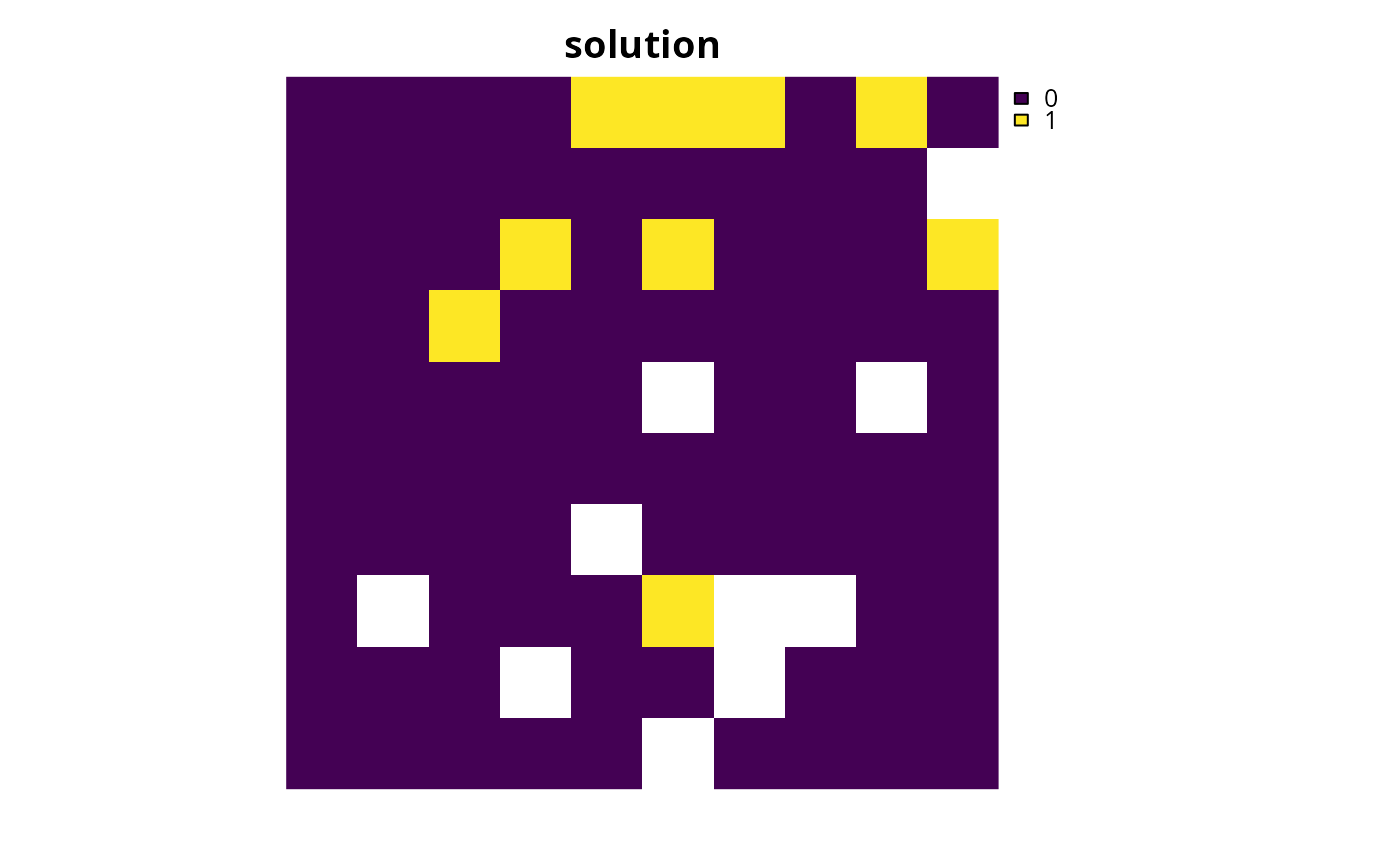# find out which features have their targets met
r4 <- eval_target_coverage_summary(p4, s4)
print(r4, width = Inf)
#> # A tibble: 5 × 9
#>   feature met   total_amount absolute_target absolute_held absolute_shortfall
#>   <chr>   <lgl>        <dbl>           <dbl>         <dbl>              <dbl>
#> 1 layer.1 FALSE         83.3            8.33          7.35              0.975
#> 2 layer.2 TRUE          31.2            3.12          3.13              0
#> 3 layer.3 FALSE         72.0            7.20          5.78              1.42
#> 4 layer.4 TRUE          42.7            4.27          4.50              0
#> 5 layer.5 FALSE         56.7            5.67          5.24              0.431
#>   relative_target relative_held relative_shortfall
#>             <dbl>         <dbl>              <dbl>
#> 1             0.1        0.0883            0.0117
#> 2             0.1        0.100             0
#> 3             0.1        0.0803            0.0197
#> 4             0.1        0.106             0
#> 5             0.1        0.0924            0.00760

# plot the example phylogeny and color the represented features in red
plot(sim_phylogeny, main = "represented features",
tip.color = replace(rep("black", nlayers(sim_features)),
which(r4$met), "red"))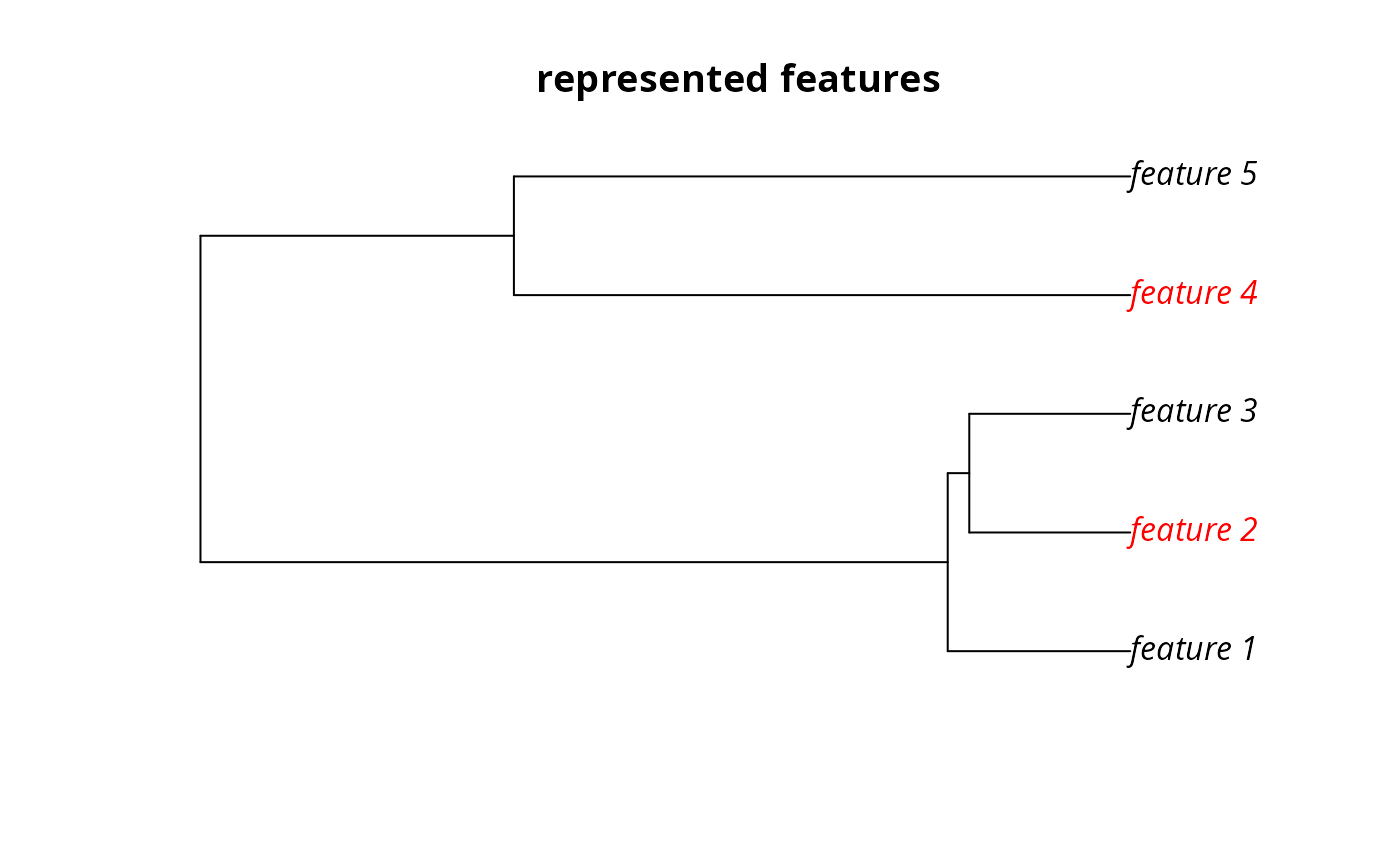# } # we can see here that the third feature ("layer.3", i.e., # sim_features[]) is not represented in the solution. Let us pretend # that it is absolutely critical this feature is adequately conserved # in the solution. For example, this feature could represent a species # that plays important role in the ecosystem, or a species that is # important commercial activities (e.g., eco-tourism). So, to generate # a solution that conserves the third feature whilst also aiming to # maximize phylogenetic diversity, we will create a set of weights that # assign a particularly high weighting to the third feature w5 <- c(0, 0, 1000, 0, 0) # we can see that this weighting (i.e., w5) has a much higher value than # the branch lengths in the phylogeny so solutions that represent this # feature be much closer to optimality print(sim_phylogeny$edge.length)
#>  1.42105185 0.34712544 0.04100098 0.30612447 0.30612447 0.59604770 1.17212960
#>  1.17212960
# \dontrun{
# create problem with high weighting for the third feature and solve it
s5 <- p4 %>% add_feature_weights(w5) %>% solve()

# plot solution
plot(s5, main = "solution", axes = FALSE, box = FALSE)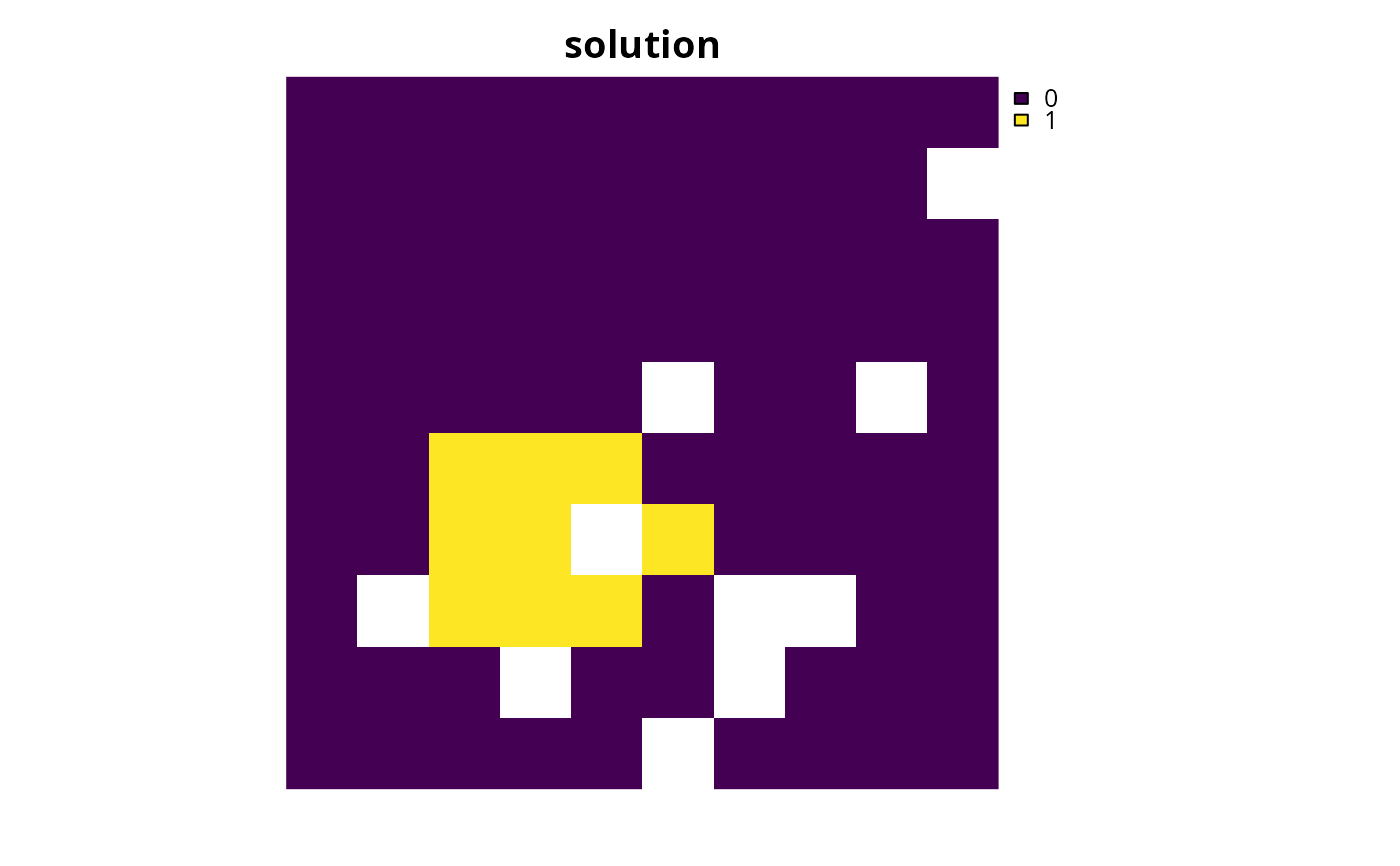# find which features have their targets met
r5 <- eval_target_coverage_summary(p4, s5)
print(r5, width = Inf)
#> # A tibble: 5 × 9
#>   feature met   total_amount absolute_target absolute_held absolute_shortfall
#>   <chr>   <lgl>        <dbl>           <dbl>         <dbl>              <dbl>
#> 1 layer.1 FALSE         83.3            8.33          7.35              0.975
#> 2 layer.2 TRUE          31.2            3.12          3.13              0
#> 3 layer.3 FALSE         72.0            7.20          5.78              1.42
#> 4 layer.4 TRUE          42.7            4.27          4.50              0
#> 5 layer.5 FALSE         56.7            5.67          5.24              0.431
#>   relative_target relative_held relative_shortfall
#>             <dbl>         <dbl>              <dbl>
#> 1             0.1        0.0883            0.0117
#> 2             0.1        0.100             0
#> 3             0.1        0.0803            0.0197
#> 4             0.1        0.106             0
#> 5             0.1        0.0924            0.00760

# plot the example phylogeny and color the represented features in red
# here we can see that this solution only adequately conserves the
# third feature. This means that, given the budget, we are faced with the
# trade-off of conserving either the third feature, or a phylogenetically
# diverse set of three different features.
plot(sim_phylogeny, main = "represented features",
tip.color = replace(rep("black", nlayers(sim_features)),
which(r5\$met), "red"))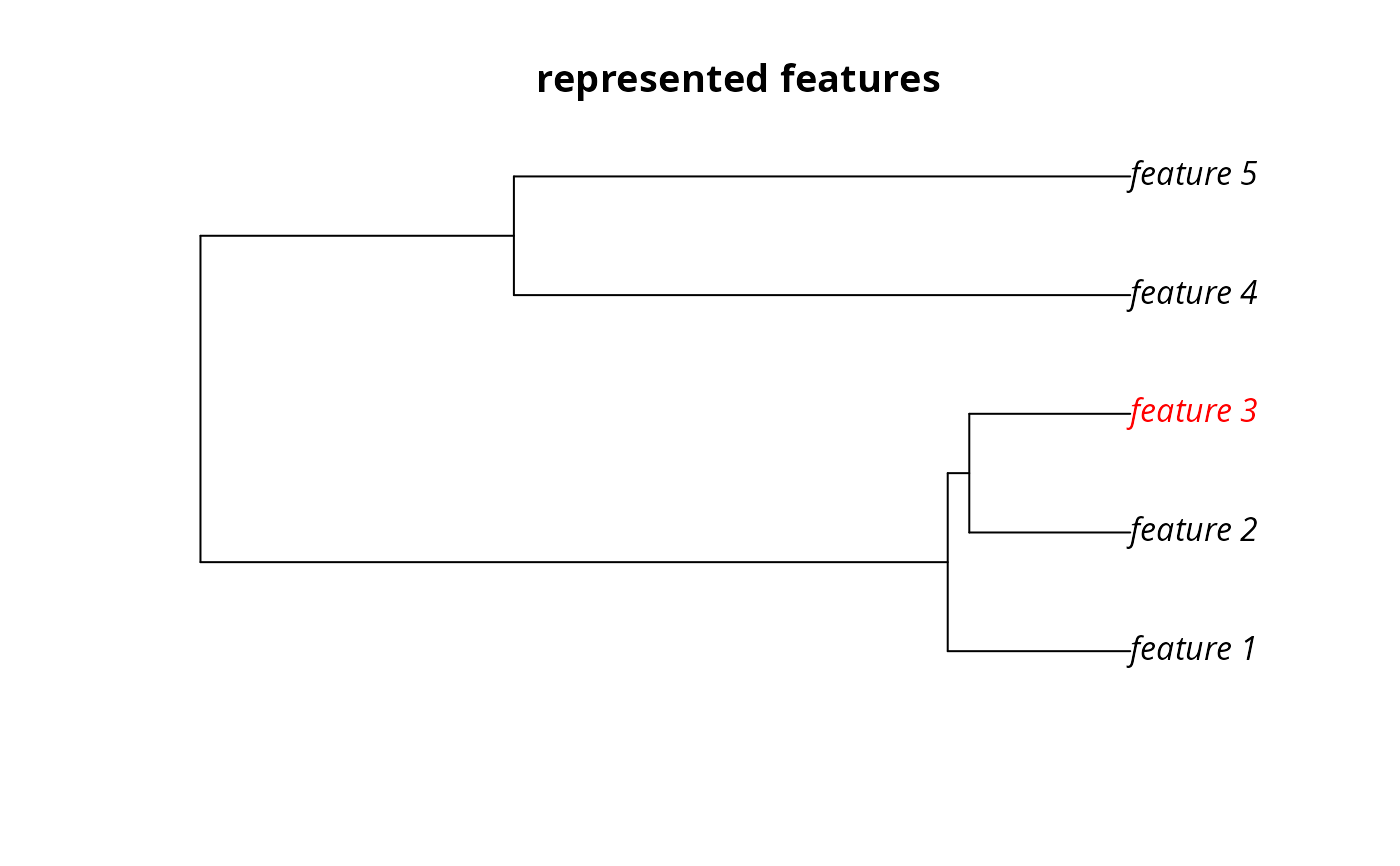# }
# create multi-zone problem with maximum features objective,
# with 10% representation targets for each feature, and set
# a budget such that the total maximum expenditure in all zones
# cannot exceed 3000
p6 <- problem(sim_pu_zones_stack, sim_features_zones) %>%
add_relative_targets(matrix(0.1, ncol = 3, nrow = 5)) %>%

# create weights that assign equal weighting for the representation
# of each feature in each zone except that it does not matter if
# feature 1 is represented in zone 1 and it really important
# that feature 3 is really in zone 1
w7 <- matrix(1, ncol = 3, nrow = 5)
w7[1, 1] <- 0
w7[3, 1] <- 100

# create problem with weights
# \dontrun{
# solve problems
s6 <- solve(p6)
s7 <- solve(p7)

# plot solutions
plot(stack(category_layer(s6), category_layer(s7)),
main = c("equal weights", "manual weights"), axes = FALSE, box = FALSE)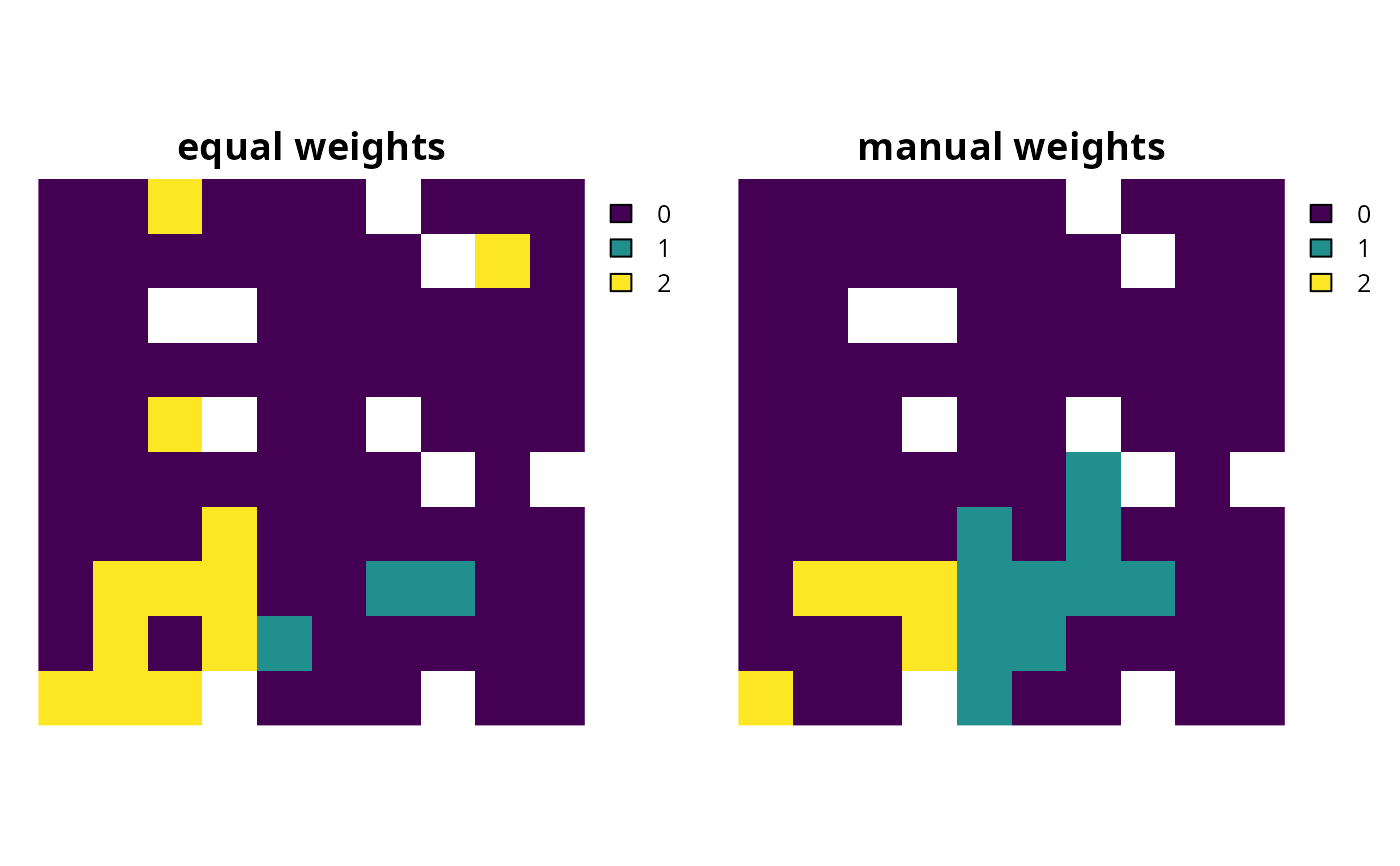# }
# create minimal problem to show the correct method for setting
# weights for problems with manual targets
p8 <- problem(sim_pu_raster, sim_features) %>%
type = "relative",
target = 0.1)) %>%
add_feature_weights(matrix(c(1, 200), ncol = 1)) %>%